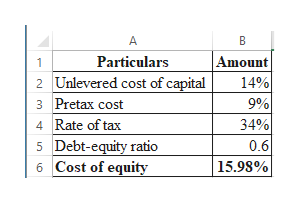# wild flowers express has a debt equity ratio of .60. the pre tax cost of debt is 9% while the unlevered cost of capital is 14%. what is the cost of levered equity if the tax rate is 34%

Question

wild flowers express has a debt equity ratio of .60. the pre tax cost of debt is 9% while the unlevered cost of capital is 14%. what is the cost of levered equity if the tax rate is 34%

check_circleExpert Solution
Step 1

Computation of levered equity cost:

Hence, the levered equity cost is 15.98%.

...help_outlineImage TranscriptioncloseAmount 14% 9% 34% Particulars 2 Unlevered cost of capital 3 Pretax cost 4 Rate of tax Debt-equity ratio Cost of equity 0.6 5 15.98% 6 fullscreen

### Want to see the full answer?

See Solution

#### Want to see this answer and more?

Solutions are written by subject experts who are available 24/7. Questions are typically answered within 1 hour*

See Solution
*Response times may vary by subject and question
Tagged in